# Given a Cumulative Distribution Function -- Find The Maximum of four Identical Distributions

#### Phovesmos

##### New member
The problem and its solution is posted below. The question that I need to ask will follow:

Problem: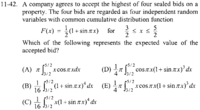Solution: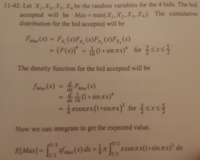My Question:

If one analyzes the multiple choice answers, one arrives at a very nice antiderivative when evaluating the Probability Density Function contained in the Expected Value for E. Indeed, this turns out to be the answer. I am not asking how to solve this problem via the process of elimination, though.

My question is as follows:

How, when evaluating max(X1, X2, X3, X4), is it possible to conclude that Fmax(x) = F(x)^4?

I understand that these events are independent and that each share a Continuous Probability Density Function. I also understand that Independent Probability Density Functions, when applied to a sequence of events, can lead to them being multiplied, but I do not recall any of this happening with the Cumulative Distribution Function, let alone what this has to do with finding the maximum of the four bids. Please help. Thanks for your guys' time.

#### Phovesmos

##### New member
For those who are interested, I've figured out the answer to this problem. I am providing the reasoning behind the solution given in the solution manual here: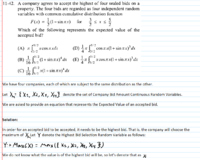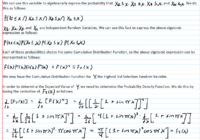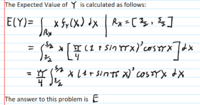My goodness, what a trip this was!

I understand that the rules state that I needed to show that I'd put in some effort, but this wasn't something for which effort could have been demonstrated. I suspect this is the reason that there was no response. After some investigating, I stumbled into a semi-explanation. Please, a little faith in me is needed!